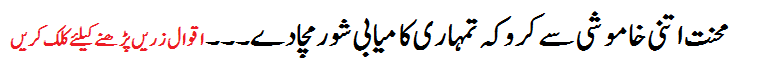# F.Sc Math Part-2 Notes On (Ch 02: Differentiation)F.Sc Part-2 (Math) Punjab Text Board
Ch No.02 (Differentiation)
exercises Of this chapter have following topics:
• Exercise No 2.1 (Average Rate of Change, Derivative of a Function)
• Exercise No 2.2 (Differentiation of Expressions of the types)
• Exercise No 2.3 (Theorems on Differentiation)
• Exercise No 2.4 (The Chain Rule, Derivatives of Inverse Function & parametric Equations & Implicit Relation)
• Exercise No 2.5 (Derivatives of Trigonometric & Inverse Trigonometric Functions)
• Exercise No 2.6 (Derivative of Exponential Functions & Logarithmic Function & Hyperbolic And Inverse Hyperbolic Function)
• Exercise No 2.7 (Successive Differentiation)
• Exercise No 2.8 (Tailor Series Expansions of Function)
• Exercise No 2.9 (Geometrical Interpretation of a Derivative, Increasing and Decreasing Function)
• Exercise No 2.10 (Relative Extrema, Critical Values and Critical Points)